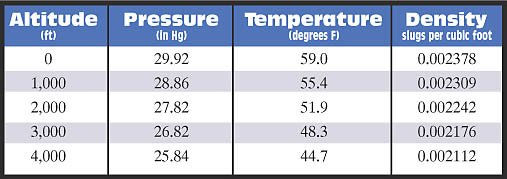top of pageSearch

# Density altitude - the secret killer

Updated: Mar 9, 2022

It's no secret that airframe icing kills pilots. It's no secret that turbulence kills pilots. It's no secret that deep, moist convection and thunderstorms kill pilots. But what about density altitude? Density altitude is perhaps just as hazardous as airframe icing and turbulence. In an older NTSB study, density altitude contributed to just as many accidents as airframe icing and as many accidents as turbulence as shown in the pie chart below.It is true that some of the density altitude accidents in this NTSB study were caused by pilots departing in an over-gross weight aircraft or using improper procedures (e.g., improper flap usage). However, pilots need to be aware that gross mistakes such as this are not forgiving when the density altitude is high.

What is density altitude? In simple terms, density altitude (DA) is pressure altitude corrected for non-standard temperature. Therefore, if the pressure and temperature throughout the atmosphere matches the standard, then pressure altitude and density altitude are the same. Of course, during the warm season, the temperature is generally above standard in most airports throughout the U.S.

While a pilot can determine pressure altitude in the cockpit (by setting the altimeter to 29.92"), there's no instrument that you can use to directly measure the density altitude. It must be calculated based on the pressure, temperature and dewpoint temperature.

The best way to think about density altitude is to first calculate the density of air (without moisture) based on a standard atmosphere. The table below is that calculation up to 4,000 feet for brevity. The column on the right is the resulting density of air based on the standard temperature and pressure while disregarding moisture (for now).Let's say you are planning to depart an airport that is located at sea level. Assuming the pressure is standard (29.92"), but the temperature is 86°F (non-standard) and the dewpoint is 64°F, what is the density altitude? If we want to utilize the table above, we first need to calculate the density of air using the temperature and dewpoint. After plugging the data into the proper equations you calculate the density of air to be 0.002242 slugs per cubic foot. Using the table above, this density equates to the same density as air at 2,000 feet.  Therefore, at sea level when the temperature is 86°F and the dewpoint is 64°F, 2,000 feet represents the density altitude for that airport.

Of course, no pilot is going to calculate the density of air while waiting to depart. This was just an exercise to understand how the actual density of air at the surface relates to a given altitude. In this particular case, the aircraft will perform as if it is at 2,000 feet MSL, not sea level.

Using this online calculator, the density altitude can be determined by simply obtaining the observed station pressure, observed station temperature and observed station dewpoint temperature. You can easily get the surface temperature and dewpoint from the ASOS or AWOS broadcast, but the surface pressure (altimeter setting) is adjusted to mean sea level. But for now, let's assume we are at sea level with standard pressure.

What about that moisture we disregarded earlier? The table above considers the atmosphere to be completely dry. Of course, that is not the case in a real atmosphere on any given day. On most warm weather days the dewpoint temperature may range from 60°F to 80°F providing plenty of moisture. The moisture in the atmosphere makes the air less dense which leads to a slightly higher density altitude.

So is there a need to correct the table above? Actually no. The correction is taken when the actual density altitude is calculated. In order to properly calculate the density altitude, virtual temperature must be used, not the actual temperature. Virtual temperature is the temperature that air will achieve if all of the moisture was removed. Many thermodynamic equations become much easier with virtual temperature since it greatly simplifies them.

The best way to visualize virtual temperature is using two parcels of air - assuming the same pressure for both parcels, of course.

Parcel One:  This air parcel has some measured temperature T1. It also has some moisture in the parcel. As a result, the parcel has a density D.

Parcel Two:  This air parcel also has density D, but has no moisture. Obviously if it has the same density with no moisture, it must have a different temperature. To achieve density D, the temperature of the second parcel must be greater than that of the first parcel. This new temperature T2 is called the virtual temperature. The virtual temperature is higher and essentially accounts for the moisture that is in the air.

Most pilots are weatherwise, but some are otherwise™

Dr. Scott Dennstaedt

Founder, EZWxBrief™

Weather Systems Engineer

CFI & former NWS research meteorologist